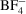International Tables for Crystallography (2006). Vol. C, ch. 9.5, pp. 790-811https://doi.org/10.1107/97809553602060000621

## Contents

• 9.5. Typical interatomic distances: organic compounds  (pp. 790-811)
• 9.5.1. Introduction  (p. 790) | html | pdf |
• 9.5.2. Methodology  (pp. 790-791) | html | pdf |
• 9.5.2.1. Selection of crystallographic data  (p. 790) | html | pdf |
• 9.5.2.2. Program system  (pp. 790-791) | html | pdf |
• 9.5.2.3. Classification of bonds  (p. 791) | html | pdf |
• 9.5.2.4. Statistics  (p. 791) | html | pdf |
• 9.5.3. Content and arrangement of the table  (pp. 791-794) | html | pdf |
• 9.5.3.1. Ordering of entries: the Bond' column  (p. 792) | html | pdf |
• 9.5.3.2. Definition of Substructure'  (pp. 792-793) | html | pdf |
• 9.5.3.3. Use of the `Note' column  (pp. 793-794) | html | pdf |
• 9.5.4. Discussion  (p. 794) | html | pdf |
• Appendix 9.5.1. Notes to Table 9.5.1.1  (p. 794) | html | pdf |
• Appendix 9.5.2. Short-form references to individual CSD entries cited by reference code in Table 9.5.1.1  (pp. 794-795) | html | pdf |
• References | html | pdf |
• Figures
• Fig. 9.5.1.1. Growth of the Cambridge Structural Database 1965–1985 as number of entries ( N ent ) published in a given year  (p. 790) | html | pdf |
• Fig. 9.5.2.1. Effect of the removal of outliers (contributors that are > 4σ from the mean) for the C—C bond in C ar —C≡N fragments  (p. 791) | html | pdf |
• Fig. 9.5.2.2. Skewed distribution of B—F bond lengths inions  (pp. 791-792) | html | pdf |
• Fig. 9.5.2.3. Resolution of the bimodal distribution of C—N bond lengths in C ar —N(C sp 3 ) 2 fragments  (p. 792) | html | pdf |
• Fig. 9.5.3.1. ( a ) Distribution of mean bond-length values reported in the table by element pair  (p. 792) | html | pdf |
• Fig. 9.5.3.2. Alphabetized index of ring systems referred to in the table; the numbering scheme used in assembling the bond-length data is given where necessary  (p. 793) | html | pdf |
• Tables
• Table 9.5.1.1. Average lengths (Å) for bonds involving the elements H, B, C, N, O, F, Si, P, S, Cl, As, Se, Br, Te, and I  (pp. 796-811) | html | pdf |